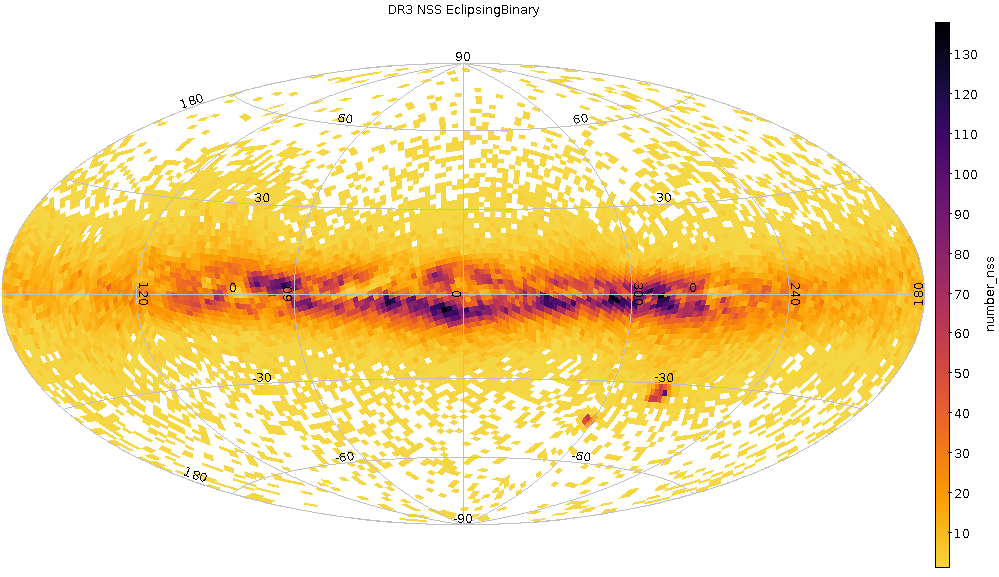# 7.7.7 General caveats

The attention of the users is drawn towards several caveats concerning the various NSS solutions and combinations.

## Incompleteness

As should be clear from Section 7.1.2, and following sections, many truncations have been done on the input data before the processing, and filtering has also been applied on its output. As for the processing itself, which starts by the detection of a variable signal, it is very much governed by the number of transit observations and their time coverage, i.e. by the inhomogeneous scanning law of the satellite. All this makes the distribution of sources, of all kind of sources, very incomplete in not easily predictable ways. For example, the sky distribution Figure 7.68 shows a larger number of solutions at the intersection between galactic plane and high ecliptic latitudes, though this does not fully represent the selection function, cf. Gaia Collaboration et al. (2023a) for a discussion.Figure 7.68: Distribution of the number off NSS solutions over the sky (galactic coordinates, HEALPix level 5): EclipsingBinary (a), SB* (b), Orbital* (c), Acceleration (d).

## Uncertainties

It may be wondered whether the formal uncertainties that are associated with all orbital parameters reasonably represent the external errors. For most nss_solution_types, these uncertainties are either the formal ones resulting from the covariance matrix (spectroscopic or eclipsing binaries) or have subsequently been reweighted to account for the observed dispersion of the residuals (astrometric binaries). In both astrometric and spectroscopic cases, it is assumed that the uncertainties can be used as is. For eclipsing binaries, however, the goodness of fit is frequently in excess and it is recommended to reweight the uncertainties using Equation 7.4.

It may also be noted that the period_error is always empty for the EclipsingBinary and EclipsingSpectro solutions, as the period does not originate from the orbital solution, having been adopted from the upstream variability analysis. The uncertainty can instead be found in input_period_error for EclipsingBinary, while for EclipsingSpectro its original value can be extracted from the vari_eclipsing_binary table using the following query (7.57):

 $\left.\begin{array}[]{l}\verb!selectVAREB.source_id,periodasperiod_nss,1./% frequencyasperiod_vareb,!\\ \verb!frequency_error/power(frequency,2)asperiod_error_vareb!\\ \verb!fromvari_eclipsing_binaryVAREB,nss_two_body_orbitNSS!\\ \verb!wherenss_solution_type=^{\prime}EclipsingSpectro^{\prime}ANDVAREB.source% _id=NSS.source_id!\end{array}\right.$ (7.57)

However it should be noted that the same restrictions as for the EclipsingBinaries from nss_two_body_orbit apply.

Only in 3 cases of EclipsingSpectro the mass ratio was also fitted and the uncertainty is then indicated. The rest of the mass ratio values are byproducts of the model selection and are not trustable. They can be identified as having no uncertainty value or by selecting solutions with bit_index equal to 1566784 or 1566848.

## Significance of semi-major axis

The $\mathrm{significance}_{\mathrm{\alpha_{0}}}$ values used in Equation 7.55 and published in the archive are calculated before the correction of the covariance matrix for the excess of the goodness-of-fit, therefore doesn’t correspond to the correct value. The correct values can be calculated offline using Equation 7.15 and Equation 7.16. Unfortunately 215 sources have $\mathrm{significance}_{\mathrm{\alpha_{0}}}<5$ and should be rejected. Additionally about 1000 sources have $\mathrm{significance}_{\mathrm{\alpha_{0}}}$ that does not exceed the value of the input solution but still are larger than 5 and should be used with caution.

## Argument of periastron

Another caveat is the following. The astrometric argument of periastron in orbital or AstroSpectroSB1 solutions as it is computed from the Thiele-Innes elements, cf. query (7.58), possess a $\pm\pi$ ambiguity since which of the two nodes is the ascending node is unknown and can be found from the radial velocity only. If the spectroscopic orbit is also known (which is the case for AstroSpectroSB1 solutions), then this ambiguity can be corrected using Equation 7.59 and the argument is unambiguously known between 0 and $2\pi$, otherwise it is computed modulo $\pi$. This is what has been done Figure 7.18, otherwise the two arguments of periastron would have appeared discrepant by $\pm\pi$ in about half of the cases.

 $\left.\begin{array}[]{l}\verb!select0.5*(atan2(b_thiele_innes-f_thiele_innes,a% _thiele_innes+g_thiele_innes)!\\ \verb!+atan2(-b_thiele_innes-f_thiele_innes,a_thiele_innes-g_thiele_innes))% asomega_ABFG,!\\ \verb!atan2(c_thiele_innes,h_thiele_innes)asomega_CH,source_id!\\ \verb!fromuser_dr3int5.nss_two_body_orbit!\\ \verb!wherenss_solution_type=^{\prime}AstroSpectroSB1^{\prime}!\\ \end{array}\right.$ (7.58)

The convention shown in Equation 7.59 can be used to align the $\omega_{\rm ABFG}$ to $\omega_{\rm CH}$.

 $\left.\begin{array}[]{l}\mathrm{If~{}}(\omega_{\rm CH}-\omega_{\rm ABFG})\%(2% \pi)>\pi/2\ :\ \omega_{\rm ABFG}=\omega_{\rm ABFG}-\pi\\ \mathrm{If~{}}(\omega_{\rm CH}-\omega_{\rm ABFG})\%(2\pi)<-\pi/2\ :\ \omega_{% \rm ABFG}=\omega_{\rm ABFG}+\pi\end{array}\right.$ (7.59)

Please refer to the appendicies of Halbwachs et al. (2023) for the computation of the Thiele-Innes elements and their associated uncertainties.

## Statistical effects

Finally, there are subtle statistical features to be accounted for, related to the transformations from Thiele-Innes elements to non-linear Campbell parameters, or to the observation distribution in the case of very low eccentricities or small signal to noise. Consequently, attention is drawn to possible misinterpretations as systematics what may actually be due to some statistical features related to the random errors. Users are referred to Gaia Collaboration et al. (2023a) for a full description of these effects.

Beside, the attention is also drawn to obvious selection effects that may preferentially detect some remaining artefacts, such as e.g. selecting the largest mass functions.

## Issues found after DR3 publication

During validation of epoch astrometry for Gaia DR4, an error was discovered, that had already had an impact on the Gaia DR3 non-single star results. The investigation showed that two specific targets suffered of this software bug. We can conclude that the solutions for Gaia DR3 4698424845771339520 (WD 0141-675; also the entry in archive table gaiadr3.binary_masses is no longer valid as it was based on the orbit solution) and Gaia DR3 5765846127180770432 (HIP 64690) both are false-positives as far as Gaia non-single star processing is concerned. Note that the astrometric and other parameters of these objects in the gaiadr3.gaia_source archive table are not affected by this issue. Investigations continue to assess if any additional sources have been impacted.